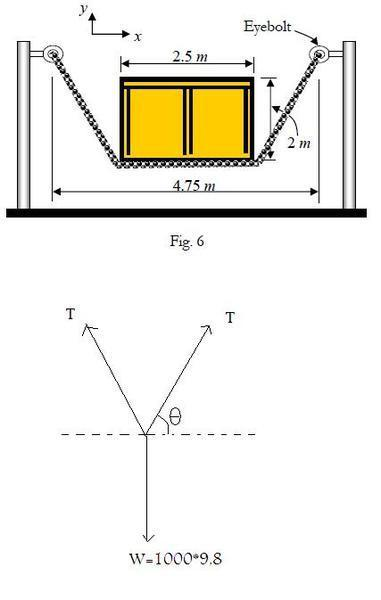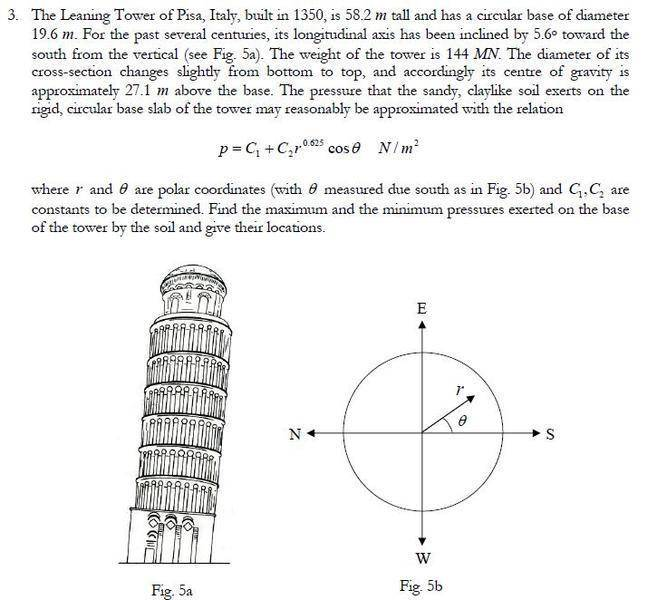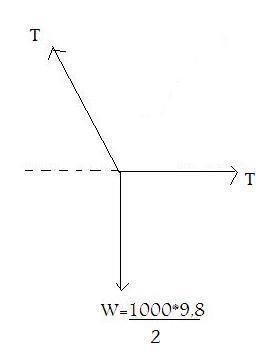# Statics of rigid body

hqjb
2 Questions about statics of rigid bodies

## Homework Statement

A cable of length 8.5 m is attached to the two posts as shown in Fig. 6. The crate has a mass of 1000 kg. Find the x and y components of the forces exerted by the cable on the eyebolts and find the tension force in the cable.## The Attempt at a Solution

$\theta = \arctan\frac{2}{1.125}$
$\theta = 60.64$
$2T\sin 60.64 = 1000 * 9.8$
$T = 5622.13N$
$T_x = 5622.13\cos 60.64 = 2756.5N$
$T_y = 5622.13\sin 60.64 = 4900.5N$

My question is regarding my free body diagram, I didn't exactly know how to draw it since the box acted its weight not at a point but along a stretch of wire.
So I drew the FBD according to an imaginary point along the centre of gravity concurrent with the cable's tension, is this correct?

## Homework Statement## The Attempt at a Solution

This one is really tough, I just need a hint on this problem. All I got was that the pressure would be minimum at r=0m(the centre) and maximum where r=19.6m and theta=0(at the south perimeter).
Not sure how I could find the constants C1 and C2.

## Answers and Replies

Mentor
My question is regarding my free body diagram, I didn't exactly know how to draw it since the box acted its weight not at a point but along a stretch of wire.
So I drew the FBD according to an imaginary point along the centre of gravity concurrent with the cable's tension, is this correct?
It works. Alternatively, you could draw two diagrams, and let 1/2 of the total force act on both sides.

All I got was that the pressure would be minimum at r=0m(the centre) and maximum where r=19.6m and theta=0(at the south perimeter).
What about the northern side, with negative cos(θ)?
Can you calculate the total force on the ground, based on C1 and C2? You have another value to compare it to, this allows to determine C1.
The center of mass has to be supported without net angular momentum. This gives a second equation which allows to determine C2.

hqjb
It works. Alternatively, you could draw two diagrams, and let 1/2 of the total force act on both sides.

What about the northern side, with negative cos(θ)?
Can you calculate the total force on the ground, based on C1 and C2? You have another value to compare it to, this allows to determine C1.
The center of mass has to be supported without net angular momentum. This gives a second equation which allows to determine C2.

-Wow thanks, didnt expect such a quick reply.
I dont understand what you mean by "let 1/2 of the total force act on both sides."
Do you mean drawing FBD of the 2 points at the edges of the crates?

-Right! Pressure is minimum at the northern side and maximum at the southern side.
So the total force would be to that the area integral of function p
i.e. $F = \int\int pdA = \int^{2\pi}_0\int^{19.6}_0 C_1 + C_2 r^{0.625}\cos\theta$
$1206.87 C_1$
For vertical equilibrium, $C_1 = 144/1206.87 MN$
Am I going in the right direction?

Mentor
-Wow thanks, didnt expect such a quick reply.
I dont understand what you mean by "let 1/2 of the total force act on both sides."
Do you mean drawing FBD of the 2 points at the edges of the crates?
What is FBD?
Right, I meant the edges of the crate.

Am I going in the right direction?
Yes

hqjb
What is FBD?
Right, I meant the edges of the crate.

Yes
Oh, its a shorthand term for freebody diagram.
A question, if I were to draw the FBD for the points at the edges it'd look like thiswhich doesn't really make sense since Tcosθ wouldn't equals T
It seems like I'm making some fundamental mistakes, forgive me if it looks stupid :shy:
Anyway, gonna sleep won't reply till tomorrow.

#### Attachments

Mentor
The force to the right can be different from T - the difference is a compressive force transported by the rigid box.
In other words, ignore the horizontal component here, it does not give anything new for your problem.

hqjb
The force to the right can be different from T - the difference is a compressive force transported by the rigid box.
In other words, ignore the horizontal component here, it does not give anything new for your problem.

I think I understand both problems already, the area integral I did above had some mistakes(namely I took diameter instead of radius and didnt multiply by the jacobian).

For the second part I took the same double integral but this time multiplied by rcosθ to get the x-component of the moments, equate this with the weight*distance and I got what hopefully is the correct answer.
Thanks.

pongo38
For the first problem I think you are missing a useful property - that the shape of the deflected cable is the same shape (to some scale) as the bending moment diagram on a beam with two point loads 2,5 m apart. What are the components in the sloping part of the cable? The vertical component must balance .... what? You know the gradient of the cable from the information given. hence you can solve for the cable force